HEJ, HU ISSN 1418-7108Manuscript no.: ANM-000926-A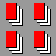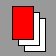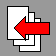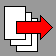# Introduction

The investigation of prime numbers has always been a very interesting research field in the history of mathematics. Through many centuries a lot of great mathematicians tried to solve the problems related to these numbers, but in spite of this there have remained even today important open questions. The theory of prime numbers plays an important role in the university mathematics education, but this field is not easy to demonstrate with the tools of traditional education. In this paper we present the most important results from prime theory and its applications in Maple. Our purpose here is not to study the whole number theory, the consideration is restricted to this specific field. Thus, sometimes we omit very important related results (such as geometrical connections), or we use some notions without deep preparation (such as congruences, number theoretical funtions). For similar reason, usually the proofs are omitted, most of these can be found in any thorough text-book, e.g. in  and . In some cases, where the proof is short and useful, it will be presented. However, there are some results too, the proof of which is extremely difficult. In these cases text-books usually refer to an original source. Through our discussion we present the short history of this field, with the names of the important authors. More historical results can be found, besides the text-books e.g. in ,  and . The illustration of theoretical results contains Maple examples and short programs. These parts suppose, that the reader has some Maple language expertise. Thorough description of the use of Maple can be found e.g. in ,  and .

Prime numbers and irreducible numbers We consider in the following integer numbers, i.e. numbers in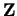. If a number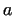is a divisor of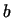, we denote it by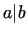.
DEFINITION 1. Prime property For a number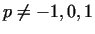let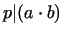. If from this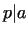or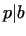follows, then we call the number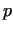a prime number.
DEFINITION 2. Irreducible property We call a numberirreducible, if fromfollows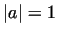or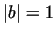.
THEOREM 1. A number is prime if and only if it is irreducible.
REMARKS 1. This theorem usually does not hold if we leave the ring. It is true in every case, that a prime element is irreducible. But the other direction gets violated in many rings. For example in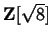the number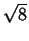is irreducible, but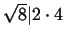does not imply, that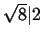or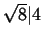. 2. Not prime elements are called composite numbers.
THEOREM 2. The base theorem of number theory Every number differ from 0, -1 and 1 can be written as the product of finite irreducible numbers. This factorization is unique regardless of the numbers 1, -1 and the rank of the factors.
In the Maple system we can factorize a number with the function ifactor.
  > ifactor(123456789012345678901234567890);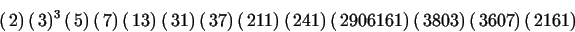HEJ, HU ISSN 1418-7108Manuscript no.: ANM-000926-A